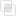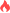Python DataFrame如何根据列值选择行

Python DataFrame2021-09-02 09:29:371502浏览 · 0收藏 · 0评论1、要选择列值等于标量的行，可以使用==。

df.loc[df['column_name'] == some_value]

2、要选择列值在可迭代中的行，可以使用isin。

df.loc[df['column_name'].isin(some_values)]

df.loc[(df['column_name'] >= A) & (df['column_name'] <= B)]

3、由于Python的运算符优先级规则，&绑定比<=and更紧密>=。

df['column_name'] >= A & df['column_name'] <= B

df['column_name'] >= (A & df['column_name']) <= Bpython默认字典是什么python命名元组如何理解python类型提示是什么python装饰器的常见用途python应用数据科学的优势python有哪些数据科学库python迭代中删除列表项目python列表如何分成大小均匀的块python函数内部修改全局变量python同名局部变量的探究python变量声明为全局变量的两种方法python global的使用限制python消除抑制警告的方法Python解释器如何读取源文件Python不同模块下特殊变量的使用js事件委托如何理解8223

js中hasOwnProperty的属性用法7368